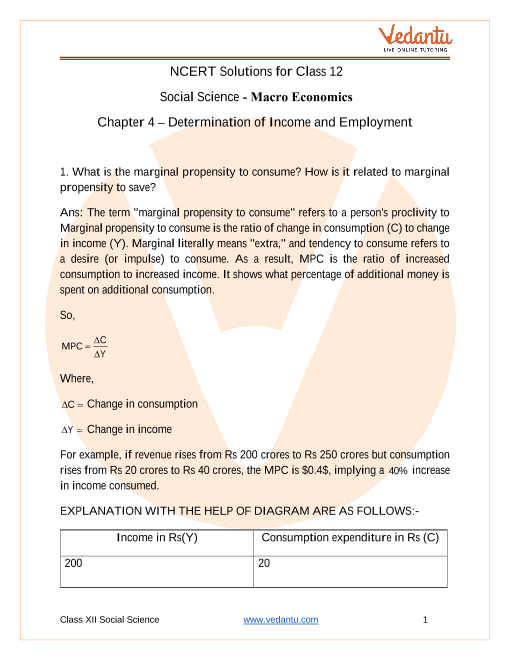# NCERT Solutions for Class 12 Macro Economics Chapter-4

## Class 12 NCERT Solutions Macro Economics - Determination of Income and Employment - Free PDF DownloadMacroeconomics Chapter 4 Class 12 deals with significant economic concepts like marginal propensity, investment, parametric shift of a line, effective demand which help the students understand crucial factors about economic determinants that affect consumer behaviour.

NCERT Class 12 Macroeconomics Chapter 4 solutions provide ideal solutions to essential questions that offer a better understanding to the students.

Do you need help with your Homework? Are you preparing for Exams?
Study without Internet (Offline)The NCERT Solutions for Class 12 Macroeconomics Chapter 4 Income Determination are readily available in downloadable PDF versions from reliable education websites like Vedantu. These solutions are curated by scholar-teachers in the specific subjects strictly adhering to updated CBSE syllabus and question pattern. The PDF versions are useful for quick references and detailed revisions of both long and short questions.

Given below are some of the Ch 4 Macroeconomics Class 12 solutions:

• Economics Class 12 Chapter 4 Macroeconomics Solution Question 1 and 2

Both these solutions help the students to understand the fundamentals of the chapter. The answer to question one comes with detailed discussion on marginal propensity to consume and its relation to marginal propensity to save with extensive illustrations.

Solution 2 clarifies the concepts of investments like Ex-ante investment and ex-post investment. The answer comes with extensive analysis and can help a student develop a better understanding of crucial concepts.

• Class 12th Macroeconomics Chapter 4 Solutions Question 3 and 4

These questions come with extensive illustrations and detailed examples with diagrams and try to clarify concepts of Parametric shift of a line and effective demand. These solutions will help the student clarify concepts like slope decrease and intercept increase adequately.

• NCERT Solutions of Chapter 4 of Macroeconomics Class 12 Question 5

Question 5 of Class 12 the NCERT solutions provides a mathematical solution to measure Ex-ante aggregate demand. The mathematical solutions are provided in a step by step manner with illustration on each step that will enable the students to understand the nuances about the question and calculations.

### Macroeconomics Chapter 4 Class 12 Marks Weightage

The question pattern consists of both long and short answer types, and the marks weightage is equally distributed in all the three question patterns. The marking scheme is framed with:

• MCQ question of 1 mark.

• Short answer type I - 3 marks.

• Short answer type II - 4 marks.

• Long answers of 6 marks.

The students, therefore, must be thorough with the chapter and the NCERT solutions to answer both short and long questions effectively.

• Explicitly curated by Expert teachers.

• Comes with detailed illustrations.

• Consists of diagrammatic solutions.

• Extensive numbers of example.

• Step by step mathematical solutions.

The students appearing for Class 12th CBSE board exam should keenly follow the NCERT solutions for detailed reference to crucial topics and essential questions.

1. What are the Important Topics and Subtopics in Macroeconomics?

Ans. The important topics and subtopics of Class 12 CBSE Macroeconomics are a detailed understanding of - Introduction to Macroeconomics, National income accounting, Money and Banking, Determination of Income and Employment, Government Budget and the Economy, Open Economy Macroeconomics.

Apart from the broader topics, the important sub-topic includes product method, expenditure method and income methods, functions of money, money created by banking systems, demand and supply, marginal propensity, parametric shifts of line, effective demands, paradox of thrifts.

All these chapters are important for both MCQs, short, very short, and long answer questions that come with equal mars weightage.

2. What are Some Crucial Questions From Class 12 Macroeconomics?

Ans. Some of the must-know essential questions from Class 12 Macroeconomics that are crucial for conceptual understanding are:

1. Explain the concept of Paradox Thrift.

2. What is the difference between Ex-ante investment and Ex-post investment?

3. Essential features of the Capitalist Economy.

4. Calculation of personal income and personal disposable income.

5. Difference between planned and unplanned inventory accumulation.

6. What is a barter system, and what are its various drawbacks?

7. Explain the concept of the Liquidity trap.

8. Explain why the government must provide a public good.

9. Distinguish between revenue and capital expenditure.

10. Difference between the balance of trade and current account balance.

3. How Helpful are the NCERT Solutions in Class 12 Macroeconomics?

Ans. The NCERT solutions to Class 12 Macroeconomics is extensively helpful for students preparing for the CBSE board exams. It covers in detail the questions from important topics like money and banking, determination of income and employment, government budget and the economy, open economy Macroeconomics.

Teachers explicitly curate these solutions. These solutions come with a detailed example, step by step illustrations and diagrams to provide a better understanding of the topics for the students. The solutions cover questions of both short answer and long answer types and appear extremely helpful for last moment preparation of complicated topics and revision.SHARETWEETSHARESUBSCRIBE